Chapter 4 Dynamics: Force and Newton’s Laws of Motion

# 24 4.3 Newton’s Second Law of Motion: Concept of a System

### Summary

• Define net force, external force, and system.
• Understand Newton’s second law of motion.
• Apply Newton’s second law to determine the weight of an object.

Newton’s second law of motion is closely related to Newton’s first law of motion. It mathematically states the cause and effect relationship between force and changes in motion. Newton’s second law of motion is more quantitative and is used extensively to calculate what happens in situations involving a force. Before we can write down Newton’s second law as a simple equation giving the exact relationship of force, mass, and acceleration, we need to sharpen some ideas that have already been mentioned.

First, what do we mean by a change in motion? The answer is that a change in motion is equivalent to a change in velocity. A change in velocity means, by definition, that there is an acceleration. Newton’s first law says that a net external force causes a change in motion; thus, we see that a net external force causes acceleration.

Another question immediately arises. What do we mean by an external force? An intuitive notion of external is correct—an external force acts from outside the system of interest. For example, in Figure 1(a) the system of interest is the wagon plus the child in it. The two forces exerted by the other children are external forces. An internal force acts between elements of the system. Again looking at Figure 1(a), the force the child in the wagon exerts to hang onto the wagon is an internal force between elements of the system of interest. Only external forces affect the motion of a system, according to Newton’s first law. (The internal forces actually cancel, as we shall see in the next section.) You must define the boundaries of the system before you can determine which forces are external. Sometimes the system is obvious, whereas other times identifying the boundaries of a system is more subtle. The concept of a system is fundamental to many areas of physics, as is the correct application of Newton’s laws. This concept will be revisited many times on our journey through physics.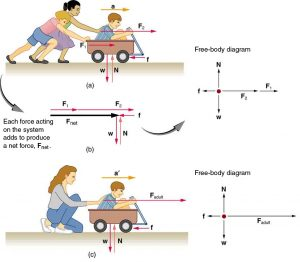Figure 1. Different forces exerted on the same mass produce different accelerations. (a) Two children push a wagon with a child in it. Arrows representing all external forces are shown. The system of interest is the wagon and its rider. The weight w of the system and the support of the ground N are also shown for completeness and are assumed to cancel. The vector f represents the friction acting on the wagon, and it acts to the left, opposing the motion of the wagon. (b) All of the external forces acting on the system add together to produce a net force, Fnet. The free-body diagram shows all of the forces acting on the system of interest. The dot represents the center of mass of the system. Each force vector extends from this dot. Because there are two forces acting to the right, we draw the vectors collinearly. (c) A larger net external force produces a larger acceleration (a’>a) when an adult pushes the child.

Now, it seems reasonable that acceleration should be directly proportional to and in the same direction as the net (total) external force acting on a system. This assumption has been verified experimentally and is illustrated in Figure 1. In part (a), a smaller force causes a smaller acceleration than the larger force illustrated in part (c). For completeness, the vertical forces are also shown; they are assumed to cancel since there is no acceleration in the vertical direction. The vertical forces are the weight$\textbf{w}$and the support of the ground$\textbf{N},$and the horizontal force$\textbf{f}$represents the force of friction. These will be discussed in more detail in later sections. For now, we will define friction as a force that opposes the motion past each other of objects that are touching. Figure 1(b) shows how vectors representing the external forces add together to produce a net force,$\textbf{F}_{\textbf{net}}.$

To obtain an equation for Newton’s second law, we first write the relationship of acceleration and net external force as the proportionality

$\boldsymbol{\textbf{a}\propto\textbf{F}_{\textbf{net}}},$

where the symbol$\boldsymbol{\propto}$means “proportional to,” and$\textbf{F}_{\textbf{net}}$is the net external force. (The net external force is the vector sum of all external forces and can be determined graphically, using the head-to-tail method, or analytically, using components. The techniques are the same as for the addition of other vectors, and are covered in Chapter 3 Two-Dimensional Kinematics.) This proportionality states what we have said in words—acceleration is directly proportional to the net external force. Once the system of interest is chosen, it is important to identify the external forces and ignore the internal ones. It is a tremendous simplification not to have to consider the numerous internal forces acting between objects within the system, such as muscular forces within the child’s body, let alone the myriad of forces between atoms in the objects, but by doing so, we can easily solve some very complex problems with only minimal error due to our simplification

Now, it also seems reasonable that acceleration should be inversely proportional to the mass of the system. In other words, the larger the mass (the inertia), the smaller the acceleration produced by a given force. And indeed, as illustrated in Figure 2, the same net external force applied to a car produces a much smaller acceleration than when applied to a basketball. The proportionality is written as

$\boldsymbol{\textbf{a}\:\propto}$$\boldsymbol{\frac{1}{m}}$

where$\boldsymbol{m}$is the mass of the system. Experiments have shown that acceleration is exactly inversely proportional to mass, just as it is exactly linearly proportional to the net external force.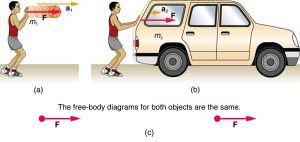It has been found that the acceleration of an object depends only on the net external force and the mass of the object. Combining the two proportionalities just given yields Newton’s second law of motion.

### NEWTON’S SECOND LAW OF MOTION

The acceleration of a system is directly proportional to and in the same direction as the net external force acting on the system, and inversely proportional to its mass.

In equation form, Newton’s second law of motion is

$\boldsymbol{\textbf{a}\:=}$$\boldsymbol{\frac{\textbf{F}_{\textbf{net}}}{m}}.$

This is often written in the more familiar form

$\boldsymbol{\textbf{F}_{\textbf{net}}=m}\textbf{a}.$

When only the magnitude of force and acceleration are considered, this equation is simply

$\boldsymbol{F_{\textbf{net}}=ma}.$
Although these last two equations are really the same, the first gives more insight into what Newton’s second law means. The law is a cause and effect relationship among three quantities that is not simply based on their definitions. The validity of the second law is completely based on experimental verification.

# Units of Force

$\boldsymbol{\textbf{F}_{\textbf{net}}=m}\textbf{a}$is used to define the units of force in terms of the three basic units for mass, length, and time. The SI unit of force is called the newton (abbreviated N) and is the force needed to accelerate a 1-kg system at the rate of$\boldsymbol{1\textbf{ m/s}^2}.$That is,$\boldsymbol{\textbf{F}_{\textbf{net}}=m}\textbf{a},$

$\boldsymbol{1\textbf{ N} = 1\textbf{ kg}\cdotp\textbf{m/s}^2}.$

While almost the entire world uses the newton for the unit of force, in the United States the most familiar unit of force is the pound (lb), where 1 N = 0.225 lb.

# Weight and the Gravitational Force

When an object is dropped, it accelerates toward the center of Earth. Newton’s second law states that a net force on an object is responsible for its acceleration. If air resistance is negligible, the net force on a falling object is the gravitational force, commonly called its weight$\textbf{w}.$Weight can be denoted as a vector$\textbf{w}$because it has a direction; down is, by definition, the direction of gravity, and hence weight is a downward force. The magnitude of weight is denoted as$\boldsymbol{w}.$Galileo was instrumental in showing that, in the absence of air resistance, all objects fall with the same acceleration$\boldsymbol{g}.$Using Galileo’s result and Newton’s second law, we can derive an equation for weight.

Consider an object with mass$\boldsymbol{m}$falling downward toward Earth. It experiences only the downward force of gravity, which has magnitude$\boldsymbol{w}.$Newton’s second law states that the magnitude of the net external force on an object is$\boldsymbol{F_{\textbf{net}}=ma}.$

Since the object experiences only the downward force of gravity,$\boldsymbol{F_{\textbf{net}}=w}.$We know that the acceleration of an object due to gravity is$\boldsymbol{g},$or$\boldsymbol{a=g}.$Substituting these into Newton’s second law gives

### WEIGHT

This is the equation for weight—the gravitational force on a mass$\boldsymbol{m}:$

$\boldsymbol{w=mg}.$

Since$\boldsymbol{g=9.80\textbf{ m/s}^2}$on Earth, the weight of a 1.0 kg object on Earth is 9.8 N, as we see:

$\boldsymbol{w=mg=(1.0\textbf{ kg})(9.80\textbf{ m/s}^2)=9.8\textbf{ N}.}$

Recall that$\boldsymbol{g}$can take a positive or negative value, depending on the positive direction in the coordinate system. Be sure to take this into consideration when solving problems with weight.

When the net external force on an object is its weight, we say that it is in free-fall. That is, the only force acting on the object is the force of gravity. In the real world, when objects fall downward toward Earth, they are never truly in free-fall because there is always some upward force from the air acting on the object.

The acceleration due to gravity$\boldsymbol{g}$varies slightly over the surface of Earth, so that the weight of an object depends on location and is not an intrinsic property of the object. Weight varies dramatically if one leaves Earth’s surface. On the Moon, for example, the acceleration due to gravity is only$\boldsymbol{1.67\textbf{ m/s}^2}.$A 1.0-kg mass thus has a weight of 9.8 N on Earth and only about 1.7 N on the Moon.

The broadest definition of weight in this sense is that the weight of an object is the gravitational force on it from the nearest large body, such as Earth, the Moon, the Sun, and so on. This is the most common and useful definition of weight in physics. It differs dramatically, however, from the definition of weight used by NASA and the popular media in relation to space travel and exploration. When they speak of “weightlessness” and “microgravity,” they are really referring to the phenomenon we call “free-fall” in physics. We shall use the above definition of weight, and we will make careful distinctions between free-fall and actual weightlessness.

It is important to be aware that weight and mass are very different physical quantities, although they are closely related. Mass is the quantity of matter (how much “stuff”) and does not vary in classical physics, whereas weight is the gravitational force and does vary depending on gravity. It is tempting to equate the two, since most of our examples take place on Earth, where the weight of an object only varies a little with the location of the object. Furthermore, the terms mass and weight are used interchangeably in everyday language; for example, our medical records often show our “weight” in kilograms, but never in the correct units of newtons.

### COMMON MISCONCEPTIONS: MASS VS. WEIGHT

Mass and weight are often used interchangeably in everyday language. However, in science, these terms are distinctly different from one another. Mass is a measure of how much matter is in an object. The typical measure of mass is the kilogram (or the “slug” in English units). Weight, on the other hand, is a measure of the force of gravity acting on an object. Weight is equal to the mass of an object ($\boldsymbol{m}$) multiplied by the acceleration due to gravity ($\boldsymbol{g}$). Like any other force, weight is measured in terms of newtons (or pounds in English units).

Assuming the mass of an object is kept intact, it will remain the same, regardless of its location. However, because weight depends on the acceleration due to gravity, the weight of an object can change when the object enters into a region with stronger or weaker gravity. For example, the acceleration due to gravity on the Moon is$\boldsymbol{1.67\textbf{ m/s}^2}$(which is much less than the acceleration due to gravity on Earth,$\boldsymbol{9.80\textbf{ m/s}^2}$). If you measured your weight on Earth and then measured your weight on the Moon, you would find that you “weigh” much less, even though you do not look any skinnier. This is because the force of gravity is weaker on the Moon. In fact, when people say that they are “losing weight,” they really mean that they are losing “mass” (which in turn causes them to weigh less).

### TAKE-HOME EXPERIMENT: MASS AND WEIGHT

What do bathroom scales measure? When you stand on a bathroom scale, what happens to the scale? It depresses slightly. The scale contains springs that compress in proportion to your weight—similar to rubber bands expanding when pulled. The springs provide a measure of your weight (for an object which is not accelerating). This is a force in newtons (or pounds). In most countries, the measurement is divided by 9.80 to give a reading in mass units of kilograms. The scale measures weight but is calibrated to provide information about mass. While standing on a bathroom scale, push down on a table next to you. What happens to the reading? Why? Would your scale measure the same “mass” on Earth as on the Moon?

### Example 1: What Acceleration Can a Person Produce when Pushing a Lawn Mower?

Suppose that the net external force (push minus friction) exerted on a lawn mower is 51 N (about 11 lb) parallel to the ground. The mass of the mower is 24 kg. What is its acceleration?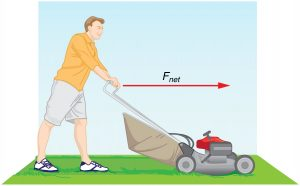Strategy

Since$\textbf{F}_{\textbf{net}}$and$\boldsymbol{m}$are given, the acceleration can be calculated directly from Newton’s second law as stated in$\boldsymbol{\textbf{F}_{\textbf{net}}=m\textbf{a}}.$

Solution

The magnitude of the acceleration$\boldsymbol{a}$is$\boldsymbol{a=\frac{F_{\textbf{net}}}{m}}.$Entering known values gives

$\boldsymbol{a\:=}$$\boldsymbol{\frac{51\textbf{ N}}{24\textbf{ kg}}}$

Substituting the units$\boldsymbol{\textbf{ kg}\cdotp\textbf{m/s}^2}$for N yields

$\boldsymbol{a\:=}$$\boldsymbol{\frac{51\textbf{ kg}\cdotp\textbf{m/s}^2}{24\textbf{ kg}}}$$\boldsymbol{=\:2.1\textbf{ m/s}^2.}$

Discussion

The direction of the acceleration is the same direction as that of the net force, which is parallel to the ground. There is no information given in this example about the individual external forces acting on the system, but we can say something about their relative magnitudes. For example, the force exerted by the person pushing the mower must be greater than the friction opposing the motion (since we know the mower moves forward), and the vertical forces must cancel if there is to be no acceleration in the vertical direction (the mower is moving only horizontally). The acceleration found is small enough to be reasonable for a person pushing a mower. Such an effort would not last too long because the person’s top speed would soon be reached.

### Example 2: What Rocket Thrust Accelerates This Sled?

Prior to manned space flights, rocket sleds were used to test aircraft, missile equipment, and physiological effects on human subjects at high speeds. They consisted of a platform that was mounted on one or two rails and propelled by several rockets. Calculate the magnitude of force exerted by each rocket, called its thrust$\textbf{T},$for the four-rocket propulsion system shown in Figure 4. The sled’s initial acceleration is$\boldsymbol{49\textbf{ m/s}^2},$the mass of the system is 2100 kg, and the force of friction opposing the motion is known to be 650 N.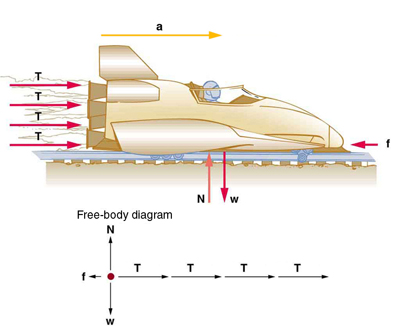Figure 4. A sled experiences a rocket thrust that accelerates it to the right. Each rocket creates an identical thrust T. As in other situations where there is only horizontal acceleration, the vertical forces cancel. The ground exerts an upward force N on the system that is equal in magnitude and opposite in direction to its weight, w. The system here is the sled, its rockets, and rider, so none of the forces between these objects are considered. The arrow representing friction (f) is drawn larger than scale.

Strategy

Although there are forces acting vertically and horizontally, we assume the vertical forces cancel since there is no vertical acceleration. This leaves us with only horizontal forces and a simpler one-dimensional problem. Directions are indicated with plus or minus signs, with right taken as the positive direction. See the free-body diagram in the figure.

Solution

Since acceleration, mass, and the force of friction are given, we start with Newton’s second law and look for ways to find the thrust of the engines. Since we have defined the direction of the force and acceleration as acting “to the right,” we need to consider only the magnitudes of these quantities in the calculations. Hence we begin with

$\boldsymbol{F_{\textbf{net}}=ma},$

where$\boldsymbol{F_{\textbf{net}}}$net is the net force along the horizontal direction. We can see from Figure 4 that the engine thrusts add, while friction opposes the thrust. In equation form, the net external force is

$\boldsymbol{F_{\textbf{net}}=4T-f}$.

Substituting this into Newton’s second law gives

$\boldsymbol{F_{\textbf{net}}=ma=4T-f}.$

Using a little algebra, we solve for the total thrust 4T:

$\boldsymbol{4\:T=ma+f}.$

Substituting known values yields

$\boldsymbol{4\:T=ma+f=(2100\textbf{ kg})(49\textbf{ m/s}^2)+650\textbf{ N}}.$

So the total thrust is

$\boldsymbol{4\:T=1.0\times10^5\textbf{ N}},$

and the individual thrusts are

$\boldsymbol{T\:=}$$\boldsymbol{\frac{1.0\times10^5\textbf{ N}}{4}}$$\boldsymbol{=\:2.6\times10^4\textbf{ N}}.$

Discussion

The numbers are quite large, so the result might surprise you. Experiments such as this were performed in the early 1960s to test the limits of human endurance and the setup designed to protect human subjects in jet fighter emergency ejections. Speeds of 1000 km/h were obtained, with accelerations of 45 g‘s. (Recall that$\boldsymbol{g},$the acceleration due to gravity, is$\boldsymbol{9.80\textbf{ m/s}^2}.$When we say that an acceleration is 45 g‘s, it is$\boldsymbol{45\times9.80\textbf{ m/s}^2},$which is approximately$\boldsymbol{440\textbf{ m/s}^2}$.) While living subjects are not used any more, land speeds of 10,000 km/h have been obtained with rocket sleds. In this example, as in the preceding one, the system of interest is obvious. We will see in later examples that choosing the system of interest is crucial—and the choice is not always obvious.

Newton’s second law of motion is more than a definition; it is a relationship among acceleration, force, and mass. It can help us make predictions. Each of those physical quantities can be defined independently, so the second law tells us something basic and universal about nature. The next section introduces the third and final law of motion.

## Section Summary

• Acceleration,$\textbf{a},$is defined as a change in velocity, meaning a change in its magnitude or direction, or both.
• An external force is one acting on a system from outside the system, as opposed to internal forces, which act between components within the system.
• Newton’s second law of motion states that the acceleration of a system is directly proportional to and in the same direction as the net external force acting on the system, and inversely proportional to its mass.
• In equation form, Newton’s second law of motion is$\boldsymbol{\textbf{a}=\frac{F_{\textbf{net}}}{m}}.$
• This is often written in the more familiar form:$\boldsymbol{F_{\textbf{net}}=m\textbf{a}}.$
• The weight$\textbf{w}$of an object is defined as the force of gravity acting on an object of mass$\boldsymbol{m}.$The object experiences an acceleration due to gravity$\textbf{g}:$
$\boldsymbol{\textbf{w}=m\textbf{g}}.$
• If the only force acting on an object is due to gravity, the object is in free fall.
• Friction is a force that opposes the motion past each other of objects that are touching.

### Conceptual Questions

1: Which statement is correct? (a) Net force causes motion. (b) Net force causes change in motion. Explain your answer and give an example.

2: Why can we neglect forces such as those holding a body together when we apply Newton’s second law of motion?

3: Explain how the choice of the “system of interest” affects which forces must be considered when applying Newton’s second law of motion.

4: Describe a situation in which the net external force on a system is not zero, yet its speed remains constant.

5: A system can have a nonzero velocity while the net external force on it is zero. Describe such a situation.

6: A rock is thrown straight up. What is the net external force acting on the rock when it is at the top of its trajectory?

7: (a) Give an example of different net external forces acting on the same system to produce different accelerations. (b) Give an example of the same net external force acting on systems of different masses, producing different accelerations. (c) What law accurately describes both effects? State it in words and as an equation.

8: If the acceleration of a system is zero, are no external forces acting on it? What about internal forces? Explain your answers.

9: If a constant, nonzero force is applied to an object, what can you say about the velocity and acceleration of the object?

10: The gravitational force on the basketball in Figure 2 is ignored. When gravity is taken into account, what is the direction of the net external force on the basketball—above horizontal, below horizontal, or still horizontal?

### Problems & Exercises

You may assume data taken from illustrations is accurate to three digits.

1: A 63.0-kg sprinter starts a race with an acceleration of$\boldsymbol{4.20\textbf{ m/s}^2}.$What is the net external force on him?

2: If the sprinter from the previous problem accelerates at that rate for 20 m, and then maintains that velocity for the remainder of the 100-m dash, what will be his time for the race?

3: A cleaner pushes a 4.50-kg laundry cart in such a way that the net external force on it is 60.0 N. Calculate the magnitude of its acceleration.

4: Since astronauts in orbit are apparently weightless, a clever method of measuring their masses is needed to monitor their mass gains or losses to adjust diets. One way to do this is to exert a known force on an astronaut and measure the acceleration produced. Suppose a net external force of 50.0 N is exerted and the astronaut’s acceleration is measured to be$\boldsymbol{0.893\textbf{ m/s}^2}.$(a) Calculate her mass. (b) By exerting a force on the astronaut, the vehicle in which they orbit experiences an equal and opposite force. Discuss how this would affect the measurement of the astronaut’s acceleration. Propose a method in which recoil of the vehicle is avoided.

5: In Figure 3, the net external force on the 24-kg mower is stated to be 51 N. If the force of friction opposing the motion is 24 N, what force$\boldsymbol{F}$(in newtons) is the person exerting on the mower? Suppose the mower is moving at 1.5 m/s when the force$\boldsymbol{F}$is removed. How far will the mower go before stopping?

6: The same rocket sled drawn in Figure 5 is decelerated at a rate of$\boldsymbol{196\textbf{ m/s}^2}.$What force is necessary to produce this deceleration? Assume that the rockets are off. The mass of the system is 2100 kg.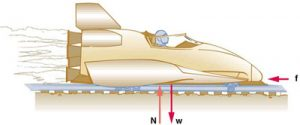Figure 5.

7: (a) If the rocket sled shown in Figure 6 starts with only one rocket burning, what is the magnitude of its acceleration? Assume that the mass of the system is 2100 kg, the thrust T is$\boldsymbol{2.4\times10^4\text{ N}},$and the force of friction opposing the motion is known to be 650 N. (b) Why is the acceleration not one-fourth of what it is with all rockets burning?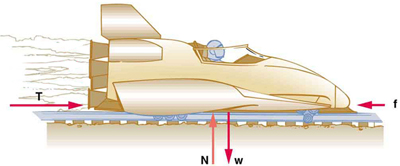Figure 6.

8: What is the deceleration of the rocket sled if it comes to rest in 1.1 s from a speed of 1000 km/h? (Such deceleration caused one test subject to black out and have temporary blindness.)

9: Suppose two children push horizontally, but in exactly opposite directions, on a third child in a wagon. The first child exerts a force of 75.0 N, the second a force of 90.0 N, friction is 12.0 N, and the mass of the third child plus wagon is 23.0 kg. (a) What is the system of interest if the acceleration of the child in the wagon is to be calculated? (b) Draw a free-body diagram, including all forces acting on the system. (c) Calculate the acceleration. (d) What would the acceleration be if friction were 15.0 N?

10: A powerful motorcycle can produce an acceleration of$\boldsymbol{3.50\textbf{ m/s}^2}$while traveling at 90.0 km/h. At that speed the forces resisting motion, including friction and air resistance, total 400 N. (Air resistance is analogous to air friction. It always opposes the motion of an object.) What is the magnitude of the force the motorcycle exerts backward on the ground to produce its acceleration if the mass of the motorcycle with rider is 245 kg?

11: The rocket sled shown in Figure 7 accelerates at a rate of$\boldsymbol{49.0\textbf{ m/s}^2}.$Its passenger has a mass of 75.0 kg. (a) Calculate the horizontal component of the force the seat exerts against his body. Compare this with his weight by using a ratio. (b) Calculate the direction and magnitude of the total force the seat exerts against his body.Figure 7.

12: Repeat the previous problem for the situation in which the rocket sled decelerates at a rate of$\boldsymbol{201\textbf{ m/s}2}.$In this problem, the forces are exerted by the seat and restraining belts.

13: The weight of an astronaut plus his space suit on the Moon is only 250 N. How much do they weigh on Earth? What is the mass on the Moon? On Earth?

14: Suppose the mass of a fully loaded module in which astronauts take off from the Moon is 10,000 kg. The thrust of its engines is 30,000 N. (a) Calculate its the magnitude of acceleration in a vertical takeoff from the Moon. (b) Could it lift off from Earth? If not, why not? If it could, calculate the magnitude of its acceleration.

## Glossary

acceleration
the rate at which an object’s velocity changes over a period of time
free-fall
a situation in which the only force acting on an object is the force due to gravity
friction
a force past each other of objects that are touching; examples include rough surfaces and air resistance
net external force
the vector sum of all external forces acting on an object or system; causes a mass to accelerate
Newton’s second law of motion
the net external force$\boldsymbol{F_{\textbf{net}}}$on an object with mass$\boldsymbol{m}$is proportional to and in the same direction as the acceleration of the object,$\textbf{a},$and inversely proportional to the mass; defined mathematically as$\boldsymbol{\textbf{a}=\frac{F_{\textbf{net}}}{m}}$
system
defined by the boundaries of an object or collection of objects being observed; all forces originating from outside of the system are considered external forces
weight
the force$\textbf{w}$due to gravity acting on an object of mass$\boldsymbol{m};$defined mathematically as:$\boldsymbol{\textbf{w}=m\textbf{g}},$where$\textbf{g}$is the magnitude and direction of the acceleration due to gravity

### Solutions

Problems & Exercises

1:

265 N

3:

$\boldsymbol{13.3\textbf{ m/s}}$

7:

(a)
$\boldsymbol{12\textbf{ m/s}^2}.$

(b) The acceleration is not one-fourth of what it was with all rockets burning because the frictional force is still as large as it was with all rockets burning.

9:

(a) The system is the child in the wagon plus the wagon.

(b)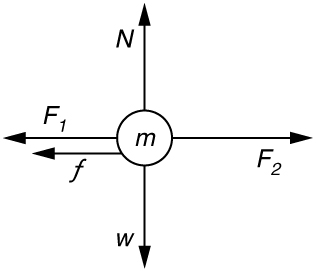Figure 8.

(c)$\boldsymbol{a=0.130\textbf{ m/s}^2}$in the direction of the second child’s push.

(d)$\boldsymbol{a=0.00\textbf{ m/s}^2}$

11:

(a)$\boldsymbol{3.68\times10^3\textbf{ N}}$. This force is 5.00 times greater than his weight.

(b)$\boldsymbol{3750\textbf{ N};\:11.3^0\textbf{ above horizontal}}$

13:

$\boldsymbol{1.5\times10^3\textbf{ N},\:150\textbf{ kg},\:150\textbf{ kg}}$

## LicenseCollege Physics: OpenStax by OpenStax is licensed under a Creative Commons Attribution 4.0 International License, except where otherwise noted.